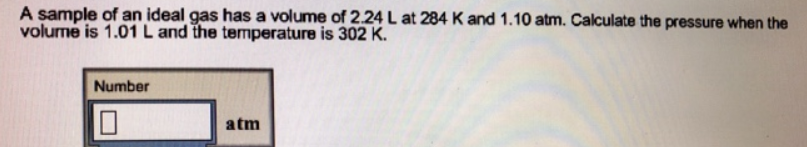# Problem: A sample of an ideal gas has a volume of 2.24 L at 284 K and 1.10 atm. Calculate the pressure when the volume is 1.01 L and the temperature is 302 K.

###### FREE Expert Solution
93% (119 ratings)###### Problem Details

A sample of an ideal gas has a volume of 2.24 L at 284 K and 1.10 atm. Calculate the pressure when the volume is 1.01 L and the temperature is 302 K.